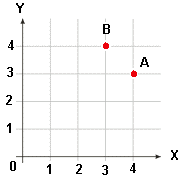HOME MATH DICTIONARY DOWNLOAD FEEDBACK DISCLAIMER
 Question: What do you mean by Coordinates ? Answer: The position of a point on a grid can be given by a pair of numbers which are its distances from two lines called the x-axis and the y-axis.A is the point (4,3). The first number 4 is called the x-coordinate. The second number 3 is called y-coordinate. The coordinates must be given in the right order. The point (3,4) is at B. Co ordinates given in this way are called Cartesian after the French mathematician Rene Descartes 1596-1650.